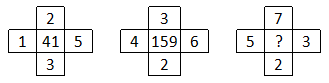Question 16

# In the following question. select the number which can be placed at the sign of question mark (?) from the given alternatives.Solution

The number in the middle is the sum of product of all the surrounding numbers and their sum.

Eg : $$(1\times2\times5\times3)+(1+2+5+3)=30+11=41$$

and $$(4\times3\times6\times2)+(4+3+6+2)=144+15=159$$

Similarly, $$(5\times7\times3\times2)+(5+7+3+2)=210+17=227$$

=> Ans - (B)

• Free SSC Study Material - 18000 Questions
• 230+ SSC previous papers with solutions PDF